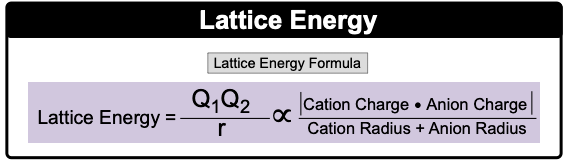Clutch Prep is now a part of Pearson
Ch.9 - Bonding & Molecular StructureWorksheetSee all chapters

# Lattice Energy

See all sections
Sections
Lewis Dot Symbols
Chemical Bonds
Dipole Moment
Octet Rule
Formal Charge
Lewis Dot Structures: Neutral Compounds
Lewis Dot Structures: Sigma & Pi Bonds
Lewis Dot Structures: Ions
Lewis Dot Structures: Exceptions
Lewis Dot Structures: Acids
Resonance Structures
Average Bond Order
Bond Energy
Coulomb's Law
Lattice Energy
Born Haber Cycle

Lattice Energy is the estimate for the strength of ionic bonds.

###### Lattice Energy

Concept #1: Lattice Energy

Lattice Formation Energy involves an exothermic process where energy is released when gaseous ions combine into ionic solid.

Example #1: The lattice formation of MgBr2 is the energy change for which one of the following processes?

Concept #2: Lattice Dissociation Energy

Lattice Dissociation Energy involves an endothermic process where energy is absorbed when ionic solid is broken up into gaseous ions.

Example #2: Which of the following reactions is associated with the lattice dissociation of Li2O?

Concept #3: Lattice Energy FormulaExample #3: Which compound possesses the strongest ionic bond: MgBr2 or KCl?

Practice: The lattice energy for ionic crystals decreases as the charge of the ions _____ and the size of the ions _____.

a) Increases, increases

b) Increases, decreases

c) Decreases, increases

d) Decreases, decreases

Concept #4: Physical Properties

Larger Lattice Energy causes ionic bonds to be stronger. This in turn causes higher boiling and melting points, but lower solubility.

Example #4: Choose the compound below that should have the highest melting point according to the ionic bonding model.

Practice: Which of the following compounds would you expect to have the highest boiling point?

a) MgCl2

b) SrO

c) SrCl2

d) CsI

e) LiBr

Practice: The solubilities of CaCrO4 and PbCrO4 in water at 25°C are approximately 0.111 g/L and 0.0905 g/L in H2O respectively. Based on this information, which compound do you think has the smaller lattice energy?